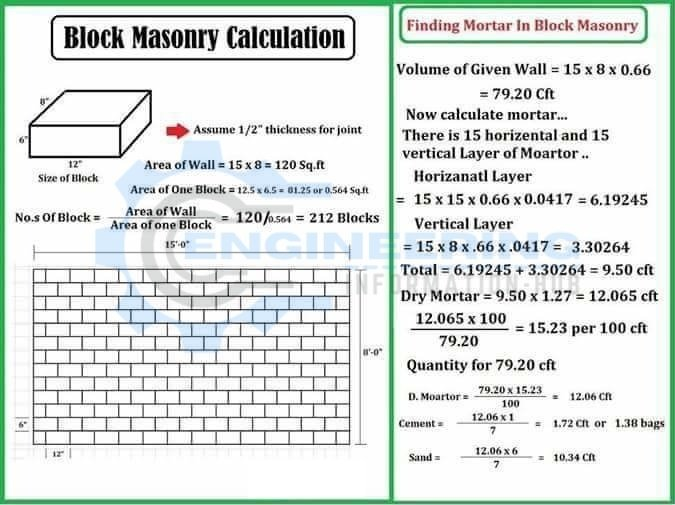# How to Calculate Block Masonry Work

### Block Masonry Calculation

In This Article, I will discuss block masonry | the calculation of block masonry | How to Calculate Block Masonry Works.

### What is The Block Masonry?

A concrete masonry unit (CMU) is a rectangular block of the standard size used to build a building. Those who use slag (ash or soil ash) as an aggregate material are called slag blocks in the United States, breeze blocks (wind is synonymous with ash) in the United Kingdom, and hollow blocks in the United States and the Philippines.

### How to Calculate Block Masonry Works

##### Calculation of Blocks Masonry:-

Assume 1/2″ thickness of Joint
Area of Wall: 15 x 8 = 120 sq.ft
Area of One Block: 12.5 x 6.5 = 81.25 or 0.564 sq.ft
No. of Blocks: Area of Wall/Area of One Block – 120/0.564 = 212 Blocks

##### Finding Mortar In Block Masonry:-

Volume of Given Wall: = 15 x 8 x 0.66 = 79.20 Cft
Calculation of Mortar: 15 horizontal and 15 vertical
Horizontal Layer: = 15 x 15 x 0.66 x 0.0417 = 6.19245
Vertical Layer: = 15 x 8 x .66 x .0417 = 3.30264
Total: = 6.19245 + 3.30264 = 9.50 cft
Dry Mortar: = 9.50 x 1.27 = 12.065 cft
12.065/79.20 x 100 = 15.23 per 100 cft
Quantity: 79.20 cft
Dry Mortar: 79.20 x 15.23/100 =12.06 cft
Cement: 12.06 x 1/7 = 1.72 cft or 1.38 bags
Sand: 12.06 x 6/7 = 10.34 cft